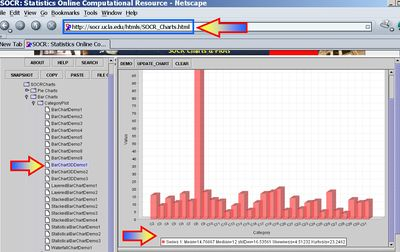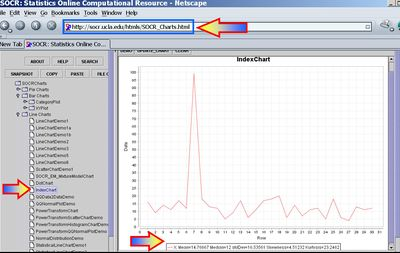# AP Statistics Curriculum 2007 Estim L Mean

## General Advance-Placement (AP) Statistics Curriculum - Estimating a Population Mean: Large Samples

This section discusses how to find point and interval estimates when the sample-sizes are large. For most applications, sample-sizes exceeding 100 may be considered as large. However, in practice even samples larger than 30 may be considered large. Yet, sometimes, samples must be > 1,000, or even 100,000 to be considered large. The next section presents the estimation for small-samples.

### Point Estimation of a Population Mean

For any process, the population mean may be estimated by a (large) sample average. That is the sample average $$\overline{X_n}={1\over n}\sum_{i=1}^n{X_i}$$, constructed from a random sample of the process {$$X_1, X_2, X_3, \cdots , X_n$$}, which is an unbiased estimate of the population mean $$\mu$$, if it exists! Note that the sample average may be susceptible to outliers.

### Definition: Confidence Interval

A type of interval that contains the true value of a parameter of interest for $$(1-\alpha)100$$% of samples taken is called a $$(1-\alpha)100$$% confidence interval for that parameter, and the ends of the CI are called confidence limits. For example, a 95% confidence interval ($$\alpha=0.05$$) for the population mean is an interval estimate (range of values) that contains the true population mean for 95% of samples taken from the same population.

### Interval Estimation of a Population Mean

For large samples, interval estimations of the population means (or Confidence Intervals) are constructed as follows. Choose a confidence level $$(1-\alpha)100%$$, where $$\alpha$$ is small (e.g., 0.1, 0.05, 0.025, 0.01, 0.001, etc.). Then a $$(1-\alpha)100%$$ confidence interval for $$\mu$$ will be $CI(\alpha): \overline{x} \pm z_{\alpha\over 2} E,$

• The Error term, E, is defined as

$$E = \begin{cases}{\sigma\over\sqrt{n}},& \texttt{for-known}-\sigma,\\ {SE},& \texttt{for-unknown}-\sigma.\end{cases}$$

• The Standard Error of the estimated $$\overline {x}$$ is obtained by replacing the unknown population standard deviation by the sample standard deviation$SE(\overline {x}) = {1\over \sqrt{n}} \sqrt{\sum_{i=1}^n{(x_i-\overline{x})^2\over n-1}}$
• Important Properties of a CI Conclusion:
• Confidence Level (alpha)
• Parameter of Interest
• Variable of Interest
• Population under study
• Confidence Interval with appropriate units

### Assumptions

The Central Limit Theorem guarantees that for large-samples, the method above provides a valid recipe for constructing a confidence interval for the population mean, no matter what the distribution of the observed data may be. Of course, for significantly non-Normal distributions, we may need to increase the sample size to guarantee that the sampling distribution of the mean is approximately Normal!

### Example

Market researchers use the number of sentences per advertisement as a measure of readability for magazine advertisements. A random sample of the number of sentences found in 30 magazine advertisements is listed. Use this sample to find point estimate for the population mean $$\mu$$. (See the data in (transposed) column format)

 16 9 14 11 17 12 99 18 13 12 5 9 17 6 11 17 18 20 6 14 7 11 12 5 18 6 4 13 11 12

A confidence interval estimate of $$\mu$$ is a range of values used to estimate a population parameter (interval estimates are normally used more than point estimates because it is very unlikely that the sample mean would match exactly with the population mean). The interval estimate uses a margin of error about the point estimate. For example if you have a point estimate of 12.25 with a margin of error of 1.75, then the interval estimate would be (10.5 to 14).

Before you find an interval estimate, you should first determine how confident you want to be that your interval estimate contains the population mean.

80% confidence (0.80), $${\alpha \over 2}=0.1$$, z = 1.28
90% confidence (0.90), $${\alpha \over 2}=0.05$$, z = 1.645
95% confidence (0.95), $${\alpha \over 2}=0.025$$, z = 1.96
99% confidence (0.99), $${\alpha \over 2}=0.005$$, z = 2.575

#### Known Variance

Suppose that we know the variance for the number of sentences per advertisement example above is known to be 256 (so the population standard deviation is $$\sigma=16$$).

• For $${\alpha \over 2}=0.1$$, the $$80% CI(\mu)$$ is constructed by:
$$\overline{x}\pm 1.28SE(\overline{x})=14.77 \pm 1.28{16\over \sqrt{30}}=[11.03;18.51]$$
• For $${\alpha \over 2}=0.05$$, the $$90% CI(\mu)$$ is constructed by:
$$\overline{x}\pm 1.645SE(\overline{x})=14.77 \pm 1.645{16\over \sqrt{30}}=[9.96;19.57]$$
• For $${\alpha \over 2}=0.005$$, the $$99% CI(\mu)$$ is constructed by:
$$\overline{x}\pm 2.575SE(\overline{x})=14.77 \pm 2.575{16\over \sqrt{30}}=[7.24;22.29]$$

Notice the increase of the CI's (directly related to the decrease of $$\alpha$$) reflecting our choice for higher confidence.

#### Unknown Variance

Suppose that we do not know the variance for the number of sentences per advertisement but use the sample variance 273 as an estimate (so the sample standard deviation is $$s=\hat{\sigma}=16.54$$).

• For $${\alpha \over 2}$$, the $$80% CI(\mu)$$ is constructed by:
$$\overline{x}\pm 1.28SE(\overline{x})=14.77 \pm 1.28{16.54\over \sqrt{30}}=[10.90;18.63]$$
• For $${\alpha \over 2}=0.05$$, the $$90% CI(\mu)$$ is constructed by:
$$\overline{x}\pm 1.645SE(\overline{x})=14.77 \pm 1.645{16.54\over \sqrt{30}}=[9.80;19.73]$$
• For $${\alpha \over 2}=0.005$$, the $$99% CI(\mu)$$ is constructed by:
$$\overline{x}\pm 2.575SE(\overline{x})=14.77 \pm 2.575{16.54\over \sqrt{30}}=[6.99;22.54]$$

Notice the increase of the CI's (directly related to the decrease of $$\alpha$$) reflecting our choice for higher confidence.

You can use the SOCR CI Analysis Applet to compute these interval estimates.

### Hands-on Activities

• See the SOCR Confidence Interval Experiment.
• Sample statistics, like the sample-mean and the sample-variance, may be easily obtained using SOCR Charts. The images below illustrate this functionality (based on the Bar-Chart and Index-Chart) using the 30 observations of the number of sentences per advertisement, reported above."-----